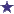# WHAT IS 2/3 OF A 45 DAY SUPPLY?

by Wondering
(Anytown, USA)Two Thirds of 45 is...

WHAT IS 2/3 OF A 45 DAY SUPPLY?

### Comments for WHAT IS 2/3 OF A 45 DAY SUPPLY?

Average RatingRating30 Days = 2/3 of a 45 Day Supply by: David 2/3 of 45 = 30 days Divide 45 by 3 = 15 multiply 15 X 2 = 30 30 days